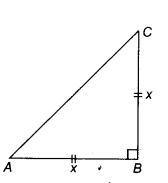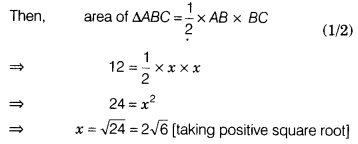# If isosceles right angled triangle has an area of 12 cm2,then find the length of its base

If an isosceles right angled triangle has an area of 12 cm2, then find the length of its base.

Let ∆ ABC is an isosceles right angled triangle having area 12 cm2 and AB = BC = x cm.Hence, length of the base of a triangle is 2√6cm